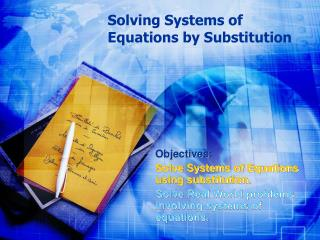DownloadDownload PresentationSolving Systems of Equations by Substitution

# Solving Systems of Equations by Substitution

Download Presentation## Solving Systems of Equations by Substitution

- - - - - - - - - - - - - - - - - - - - - - - - - - - E N D - - - - - - - - - - - - - - - - - - - - - - - - - - -
##### Presentation Transcript

1. Solving Systems of Equations by Substitution Objectives: Solve Systems of Equations using substitution. Solve Real World problems involving systems of equations.

2. Systems of Equations Review • How many ways can two lines lie on a coordinate plane? • What is the solution for a system of equations in each case?

3. Substitution • Solve each system of equations and state the solution(s) if solution(s) exist. Y = 5xand 2x +3y = 34 2x+3( ) = 34 2x + 15x = 34 17x = 34 17 x = 2 5x Y = 5( ) Y = 10 2 Solution =(2, 10)

4. Substitution • Solve each system of equations and state the solution(s) if solution(s) exist. X + y = 16 and 2y = 2x + 2 y = 16 - x 2( ) = 2x + 2 32 – 2x = 2x + 2 32 = 4x + 2 30 = 4x 7.5 = x 16 - x

5. Substitution • Solve each system of equations and state the solution(s) if solution(s) exist. X + 3y = 12 and x – y = 8

6. Substitution • Solve each system of equations and state the solution(s) if solution(s) exist. Y= 3/5 x and 3x – 5y = 15

7. Substitution • Solve each system of equations and state the solution(s) if solution(s) exist. Y=3x+2 and 6x -2y = -4

8. Substitution • C – 5d = 2 and 2c + d = 4

9. Substitution • 5r – s = 5 and -4r + 5s = 17

10. Assignment • Page 379 #11-17 • SHOW WORK!!! • Remember Solution is an ordered pair.

11. Second Page

12. Second Page